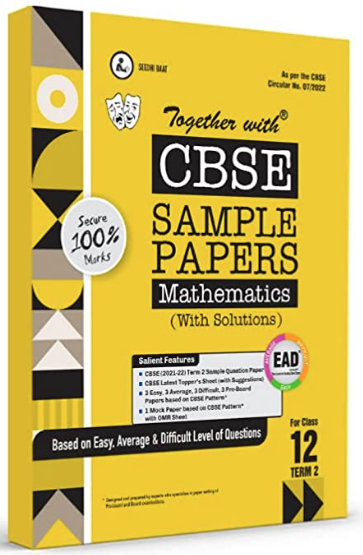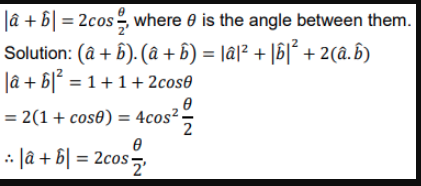# If 𝑎̂ and 𝑏̂ are unit vectors, then prove that |𝑎̂ + 𝑏̂| = 2𝑐𝑜𝑠 0/2 , where 𝜃 is the angle between them.

CBSE Sample Question Paper, Class 12 Mathematics Term 2 Question - If 𝑎̂ and 𝑏̂ are unit vectors, then prove that |𝑎̂ + 𝑏̂| = 2𝑐𝑜𝑠 0/2 , where 𝜃 is the angle between them.## If 𝑎̂ and 𝑏̂ are unit vectors, then prove that |𝑎̂ + 𝑏̂| = 2𝑐𝑜𝑠 0/2 , where 𝜃 is the angle between them.

### Answer. If 𝑎̂ and 𝑏̂ are unit vectors, then prove that